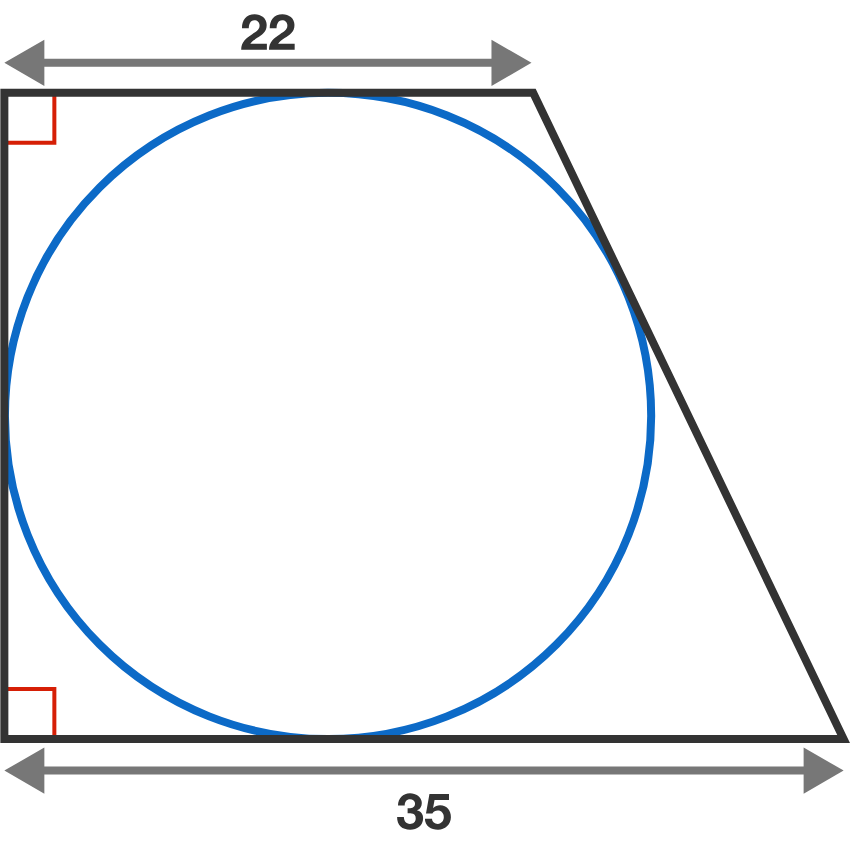# Problems of the Week

Contribute a problem

# 2018-09-17 Intermediate

A number of the form $10^n-1=\underbrace{9999...9}_{n\text{ times}},$ where $n$ is a positive integer, will never be divisible by $2$ or $5.$

Are there any other prime numbers that numbers of this form are never divisible by?

A circle is inscribed in a right trapezoid with base lengths 22 and 35.

What is the area of this trapezoid?\LARGE \begin{aligned}\large \sqrt{2^{\sqrt{2^{\sqrt{2^{\sqrt {2^{\sqrt 2}}}}}}}} = \sqrt 2^{\sqrt 2^{\sqrt 2^{\sqrt 2^{\sqrt 2} }} } < 2\end{aligned}

Is this true?

Bonus: Generalize the following. ${\LARGE \underbrace{\sqrt{x^{\sqrt{x^{\sqrt{x^{\sqrt {\cdots^{\sqrt x }}}}}}}}}_{n \text{ times}}}$

A regular decagon is obtained by joining 10 regular pentagons side by side.

Generalizing this, we claim that there is a regular $n$-gon obtained by joining $n$ regular $k$-gons side by side.

What is the sum of all possible values of $k \ge 5?$A casino owner invents a new game where a player flips a fair coin $n$ times in a row. If the player does not flip two heads in a row at any point in the $n$ flips, then he wins the game; otherwise the house wins.

To make the game popular, the casino owner wants to maximize the player's chance of winning. However, the casino needs to make a profit, so the house must win more than half of the games played over the long run.

What should the casino owner set $n$ to be?

×